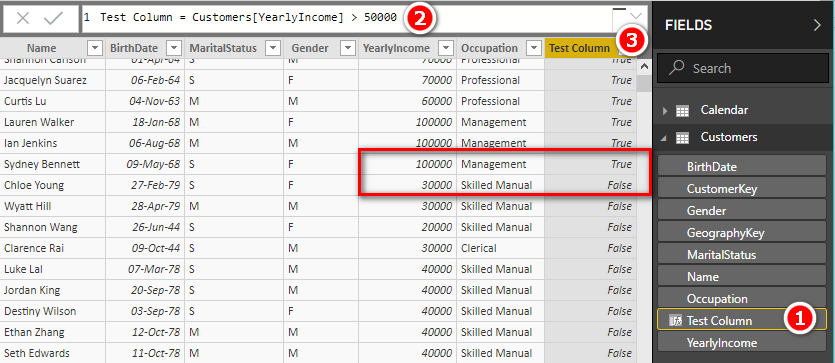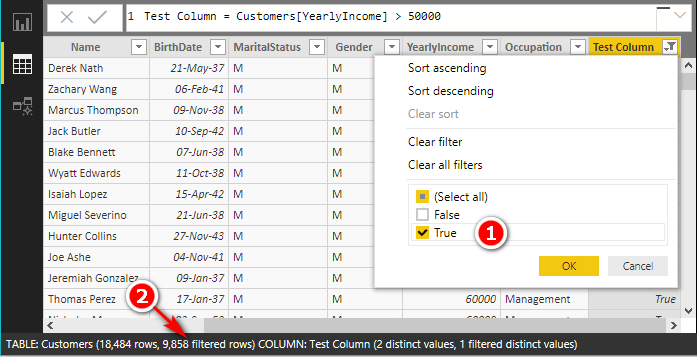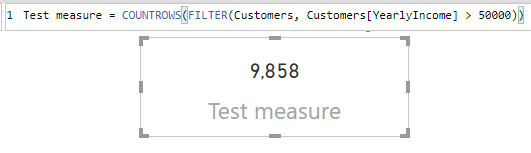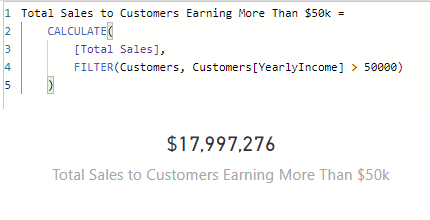The FILTER Function in DAX - Excelerator BI

# The FILTER Function in DAX

Level: Intermediate

FILTER() is one of the most important functions in the DAX language. It is quite a simple function and yet many people are scared off when they see it. In this article I will explain the basics of using the FILTER() function in DAX.  Make sure you then read the follow up article I will post next week.

## FILTER() is a Table Function

There are two types of functions in DAX, Scalar functions and Table functions.  FILTER() is the later:

### Scalar Functions

Scalar functions are functions that return a single value like a number, text, a date etc.

• A scalar is a single value
• A scalar returned from a measure can be used in the values section of a visual in Power BI and pivot tables in Excel.
• Examples include SUM(), MIN(), AVERAGE(). All these functions return a single value (scalar) as a result.

### Table Functions

Table functions are functions that return a table of values.

• Table functions cannot be used in the value section of a visual in Power BI or pivot tables in Excel.  Why?  Because the values section can only display a single value, and a table is not a single value.
• Table functions can be used anywhere that a table can be used, including
• In  DAX queries
• The “New Table” feature in Power BI
• As an input to another DAX formula regardless if that DAX formula returns a table or a scalar value.## Common Uses of the FILTER() Function

FILTER() is most often used as a filter parameter within the CALCULATE() function. OK, so now it is getting confusing. FILTER() is a function. There is another use of the word filter in DAX, and that is as a parameter in CALCULATE(). Let’s look at the syntax

`FILTER Syntax: FILTER(<table>, <true/false test>)`
`CALCULATE Syntax: CALCULATE (<measure expression>, <filter parameter>, <filter parameter>,…)`

As shown above, the CALCULATE() function can take one or more filter parameters, but those filter parameters may or may not be FILTER() functions. Don’t confuse the filter parameters inside CALCULATE() with the FILTER() function – they are two separate things. Now that we have sorted that out, I am going to use the FILTER() function as a filter parameter inside CALCULATE() in this case – this is one of the most common use cases for the FILTER() function.## Two Different Types of CALCULATE Filter Parameters

I mentioned above that CALCULATE() can take filter parameters.  There are 2 ways you can add filter parameters inside CALCULATE():

• As a simple filter.  Table[Column] compared to some value, eg
• Calendar[Year] = 2019
• Product[Category]=”Accessories”
• As a table filter:
• A table function is added as the filter parameter. The table function is evaluated first, and the results in that table are used as a filter inside CALCULATE(). eg
• FILTER(Customers, Customers[Income] > 50000)
• ALL(Products)

For this article, I am only going to be referring to table filter parameters.  I will talk more about the simple filter parameters next week.

## Understanding How FILTER() function in DAX Works

The easiest way to learn how FILTER() works is to look at an example. Below I have a measure that calculates the total sales from customers with income over \$50,000.

```Total Sales to Cust earning more than 50k =
CALCULATE(
[Total Sales],
FILTER(Customers, Customers[YearlyIncome] > 50000)
)```

### FILTER() is just like a calculated column, but better

To understand what the FILTER() function does, the easiest thing to do is to simulate what it is doing by creating a test calculated column. Those of you that have been following me for some time would know that I am not a big fan of calculated columns. But I am a big fan of using calculated columns to help get your head around what is happening under the hood.

In the image below (in the data view), I have created a new calculated column (shown as 1) in the customers table .  A calculated column has a row context.  That is just a fancy way of saying that you write a single formula and that formula (2 below) is evaluated for every row in the table, one row at a time.  The formula checks if each customer has a yearly income greater than 50,000 or not. If yes, it returns TRUE, if not it returns FALSE (3).Now that I have this column, I can manually apply a filter to this column from the data view to retain the TRUE values, as shown below.After applying this manual filter (1 above), the customers table is filtered for customers that pass the test, returning 9,858 customers (shown as 2 above).

Note: filtering a table in the data view like shown above is just for testing purposes. It has no impact on the results in your visuals. Also, if you write test columns, you should delete them when you are done.

What I have just shown you above with a calculated column is virtually identical to how the FILTER() function  in DAX works. Here is the original example formula again

```Total Sales to Cust earning more than 50k =
CALCULATE([
Total Sales],
FILTER(Customers, Customers[YearlyIncome] > 50000)
)```

Referring to just the FILTER() portion In the formula above, FILTER() is effectively (not actually) going to the customers table and adding a calculated column and then filtering the result keeping only the values that are TRUE. It ends up effectively filtering the customers table same way as the test calculated column.  FILTER() has a row context (just like a calculated column) and hence that formula is evaluated one row at a time for every row in the table specified.

One SUPER benefit of FILTER() over a calculated column is that it is all done in memory without materialising any new columns of data into your data model. The FILTER() function above is essentially creating a new filtered copy of the Customers table that only contains the customers that earn more than 50,000 per year. All virtual tables like this created in DAX will retain an active relationship to the rest of the tables in the model. This is called data lineage. All this means in practice is that any virtual table created in DAX will filter the rest of the data model in the same way that a physical table would operate.  You can read more about lineage in this article.

To show you that the result of the FILTER() function above is the same as the calculated column, let me write a test measure this time. But wait, I can’t write the following as a measure:

`Test measure = FILTER(Customers, Customers[YearlyIncome] > 50000)`

This measure above is not a legal DAX measure formula, as FILTER() returns a table, and you can’t put a table in the values section of Power BI or a pivot table in Excel. But what I CAN do is count how many rows there are in this table. If I count the rows, I can check if I get the same answer as before (9,858).

So this is the correct test measure

```Test measure =
COUNTROWS(
FILTER(
Customers,
Customers[YearlyIncome] > 50000
)
)```

When I write this test measure, you can see that I get the same result as before.Now that I know the FILTER() portion is working, I can now write my final measure## More in my Next Article

There are quite a few more things you should know and learn as you strive to understand FILTER().  Read my next article for the rest of the FILTER() story.

### 13 thoughts on “The FILTER Function in DAX”

1.Hello PowerBi support i have following dataset and i have created one calender table by using start date and end date. i puted calender table date column in slicer i want if i select 1/1/2020 (start date) and 16/1/2020 (End date) in Slicer then latest row data should be shown between selected date seprated by  order id :
my output will look like one: Output

start date end date order id amount
4/1/2020 13/1/2020 1 200
8/1/2020 12/1/2020 2 700
6/1/2020 16/1/2020 3 300

INPUT TABLE DATA

start date    end date     order id  amount

1/1/2020 10/1/2020 1 500
2/1/2020 12/1/2020 1 100
4/1/2020 13/1/2020 1 200
7/1/2020 11/1/2020 2 300
8/1/2020 12/1/2020 2 700
5/1/2020 15/1/2020 3 150
6/1/2020 16/1/2020 3 300
7/1/2020 17/2020 3 450
10/1/2020 11/1/2020 4 350

2.good article

3.Your “Supercharge PowerBI” book has very detailed explanation on this topic, and it makes every sense to me when I read this article. Very handy to have the book!

1.🙂

4.Hi Matt, just a thought.
A way to categorize filters used in CALCULATE is to separate them into open filters and closed filters. Open filters are filters that themselves are being filtered. Closed filters are ignoring the current filter context and are not themselves being filtered. Closed filters are also called simple filters. The most common simple filters have syntax shortcuts defined on them. The query analyzer loves closed/simple filters because they don’t require extra interpretation. Simple filters are immune to bidirectional filtering, another advantage.

The DAX expression you use in the blog is an open filter:

(1)
CALCULATE (
[Sales Amount],
FILTER ( Customer, Customer[Yearly Income] > 50000 )
)

However, it can be replaced with a simple filter that returns the same result and can be 2-3 times as fast depending on how it is used: (here using the syntax shortcut)

(2)
CALCULATE ( [Sales Amount], KEEPFILTERS ( Customer[Yearly Income] > 50000 ) )

An open filter can sometimes be modified into a closed filter that returns the same result:

(3)
CALCULATE (
[Sales Amount],
KEEPFILTERS (
FILTER (
ALL ( Customer ),
Customer[Yearly Income] > 50000
)
)
)

All the 3 measures will return the same result; however, (2) and (3) will be faster.

If you run the following query in DAX studio (From adventureworks DB) you can see/measure the results by commenting out the individual measures and run them one by one. The query simulates a pivot table including subtotals with the columns Occupation and Income level on rows and years on columns.

EVALUATE
SUMMARIZECOLUMNS (
Customer[Yearly Income], “Income level”
),
ROLLUPADDISSUBTOTAL ( ‘Date'[Calendar Year], “Year sub” ),
“open”, CALCULATE (
[Sales Amount],
FILTER (
Customer,
Customer[Yearly Income] > 50000
)
)
,
“simple I”, CALCULATE (
[Sales Amount],
KEEPFILTERS ( Customer[Yearly Income] > 50000 )
)
,
“simple II”, CALCULATE (
[Sales Amount],
KEEPFILTERS (
FILTER (
ALL ( Customer ),
Customer[Yearly Income] > 50000
)
)
)
)

Jes.

5.@Bruno…yeah, that quote has been stalking me, too!

6.Krishna Mysore

Excellent article Matt. When I commenced my DAX Journey, I initially struggled a lot to get a clarity on the difference between Filter parameter Vs Filter function. You have clearly articulated and I’m sure many out there will surely gain a clearly understanding of the topic. Thanks again and look forward for the second part of the topic .

1.This will be in my next article.

7.Great article Matt; I’ve recently been getting my hands dirty with the FILTER function and I think this explanation and supporting examples do a great job demystifying it while also showing how it can be audited effectively.

Nice work.

1.My original idea was to make this a much more comprehensive article, but it turned out to be a lot more work than I expected. I will probably do a part 2 article, so keep and eye out

8.Yes, but in reality is a table with the minimum number of columns. Filter parameters always are tables.

9.Great explanation of the filter function Matt. Thanks

10.Your point is correct and valid. I did try to avoid using that term, but I agree at some point you do need to understand this. As an intermediate post, I should have said so. Thanks for the suggestion

Scroll to Top